# RD Sharma Solutions for Class 8 Chapter 9 Linear Equation in One Variable Exercise 9.2

### RD Sharma Class 8 Solutions Chapter 9 Ex 9.2 PDF Free Download

The concept of transposition (transposing a term from one side to the other side, we mean changing its sign and carrying it to the other side) is discussed in Exercise 9.2 of Chapter 9. We shall also see problems based on transposition method for solving linear equations in one variable. Our experts have derived the solutions in a step by step format, which helps students understand the concepts clearly. Detailed solutions can be accessed at RD Sharma Solutions which are designed by our tutors at BYJU’S. Students can easily download the pdf from the links given below.

## Download the pdf of RD Sharma for Class 8 Maths Exercise 9.2 Chapter 9 Linear Equation in One Variable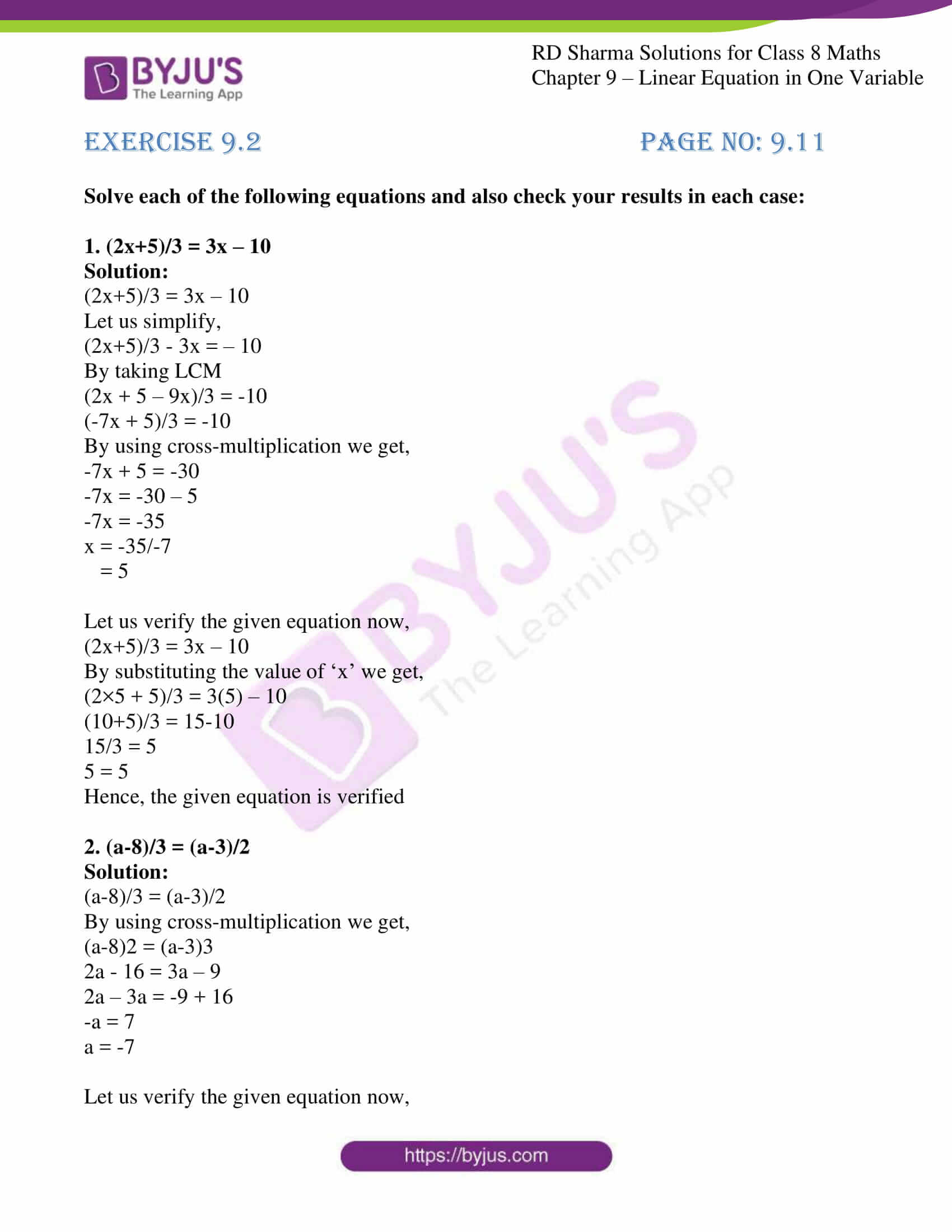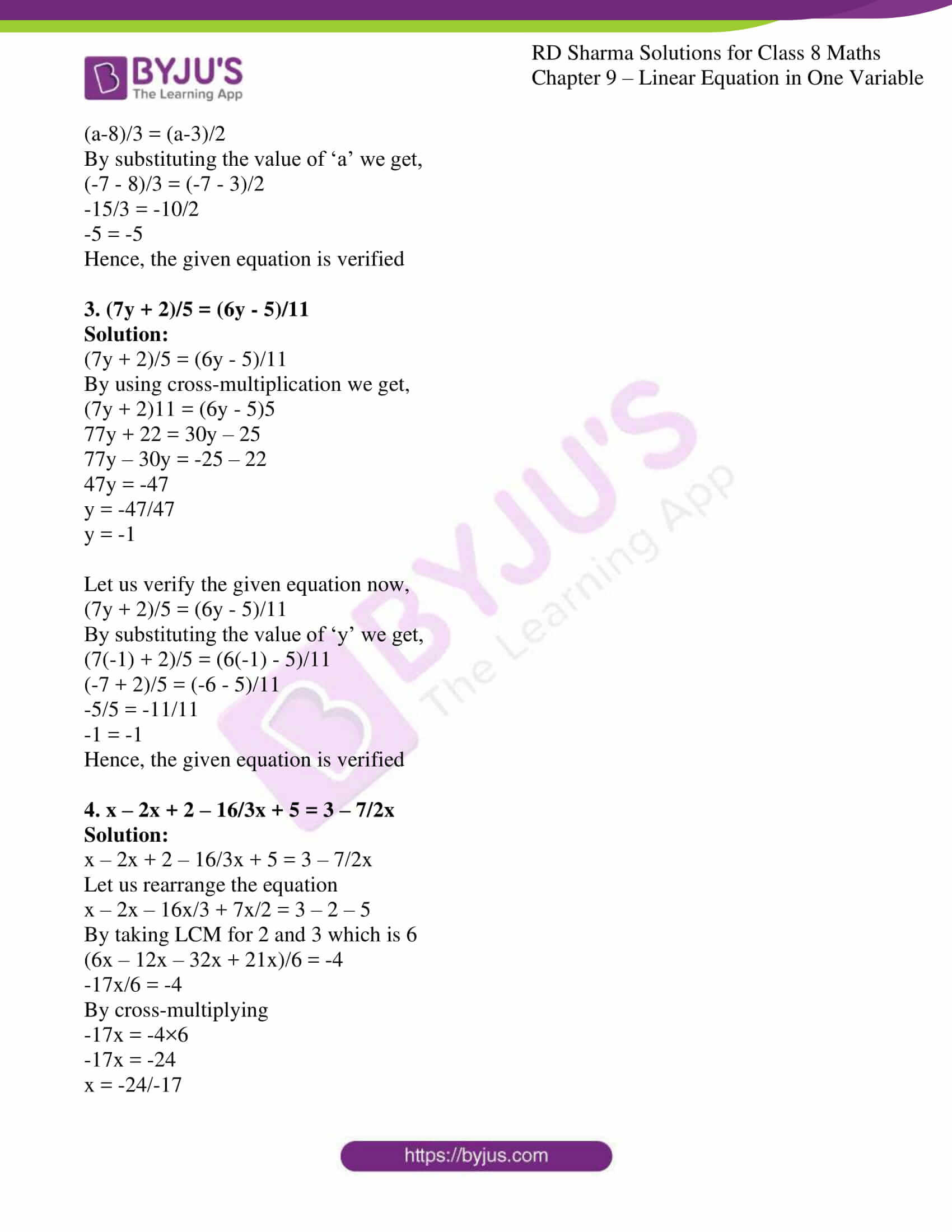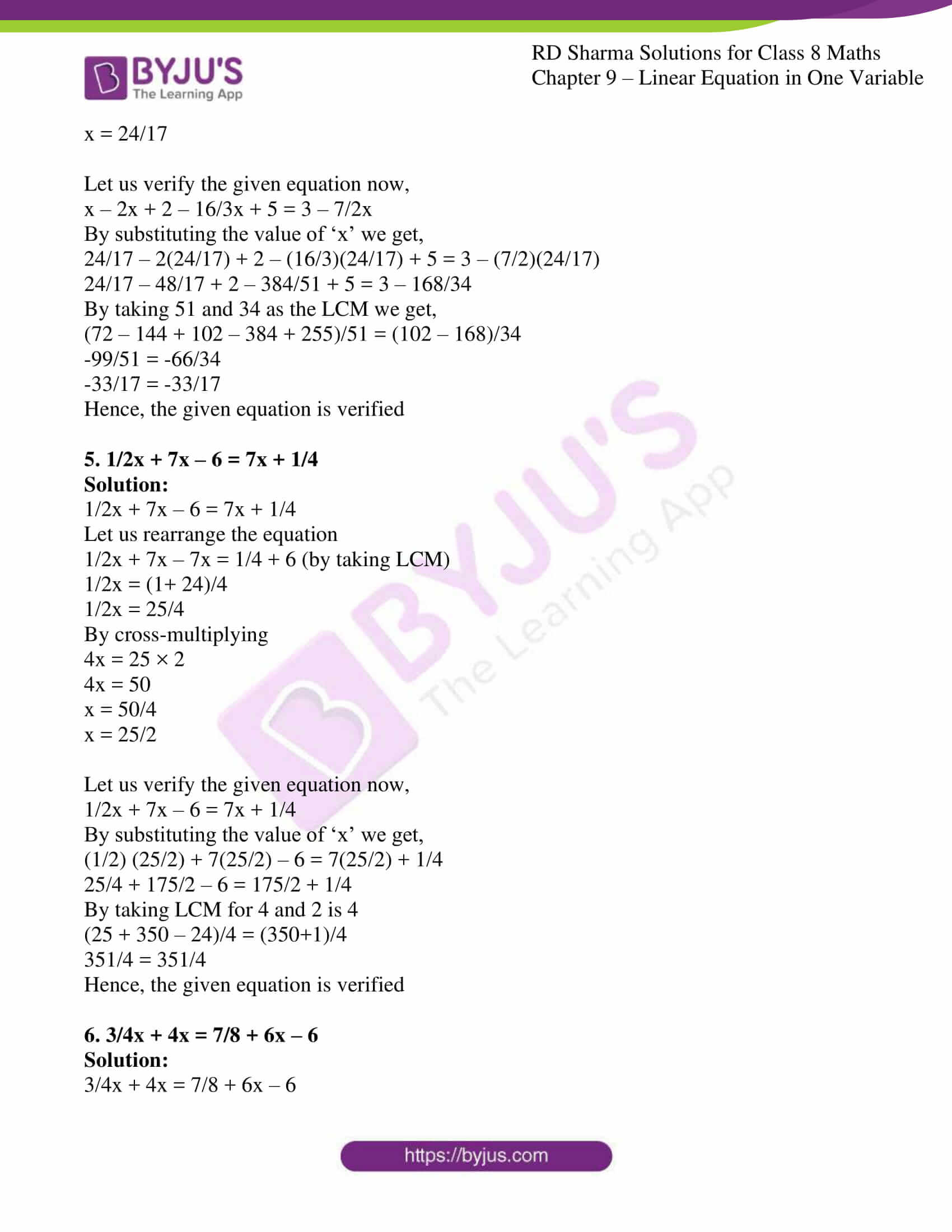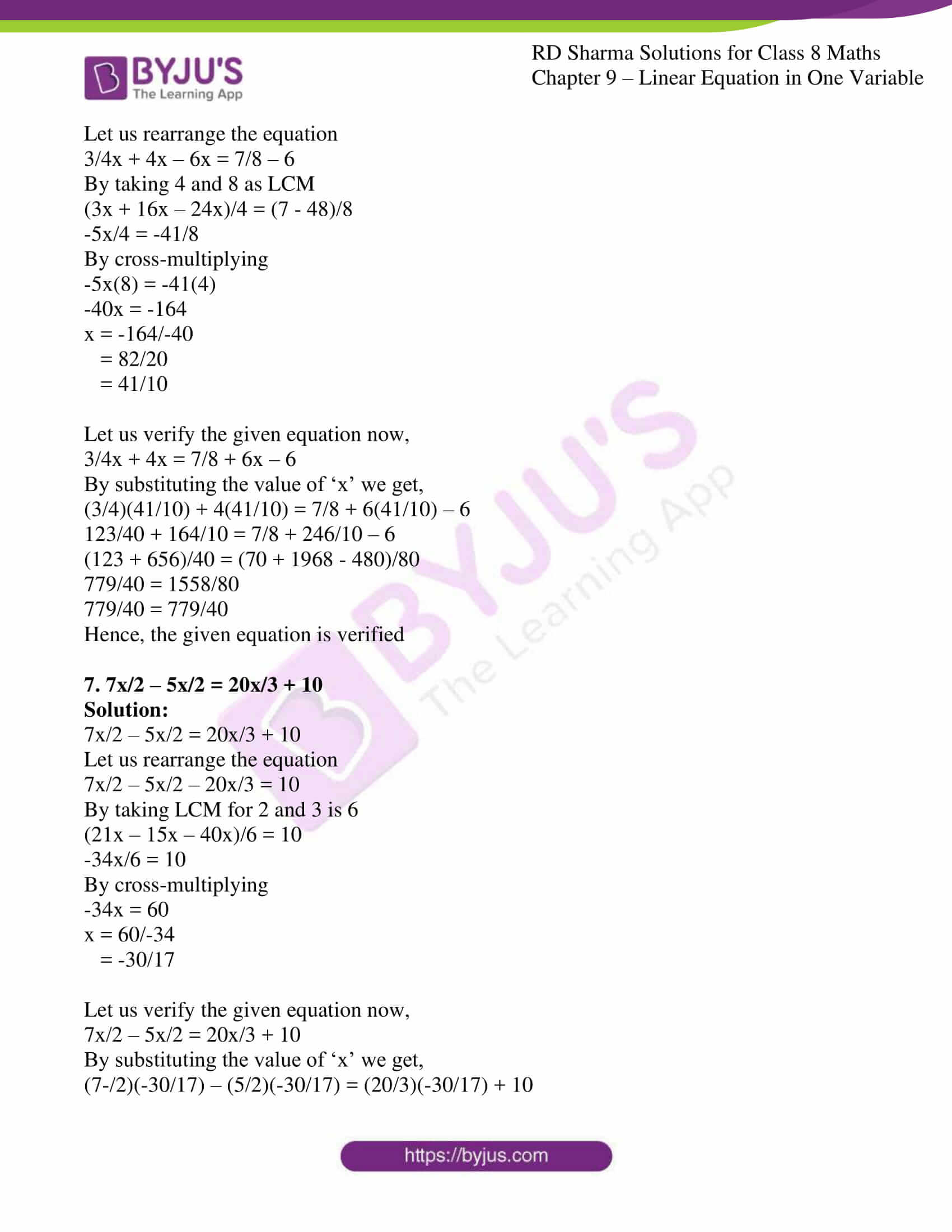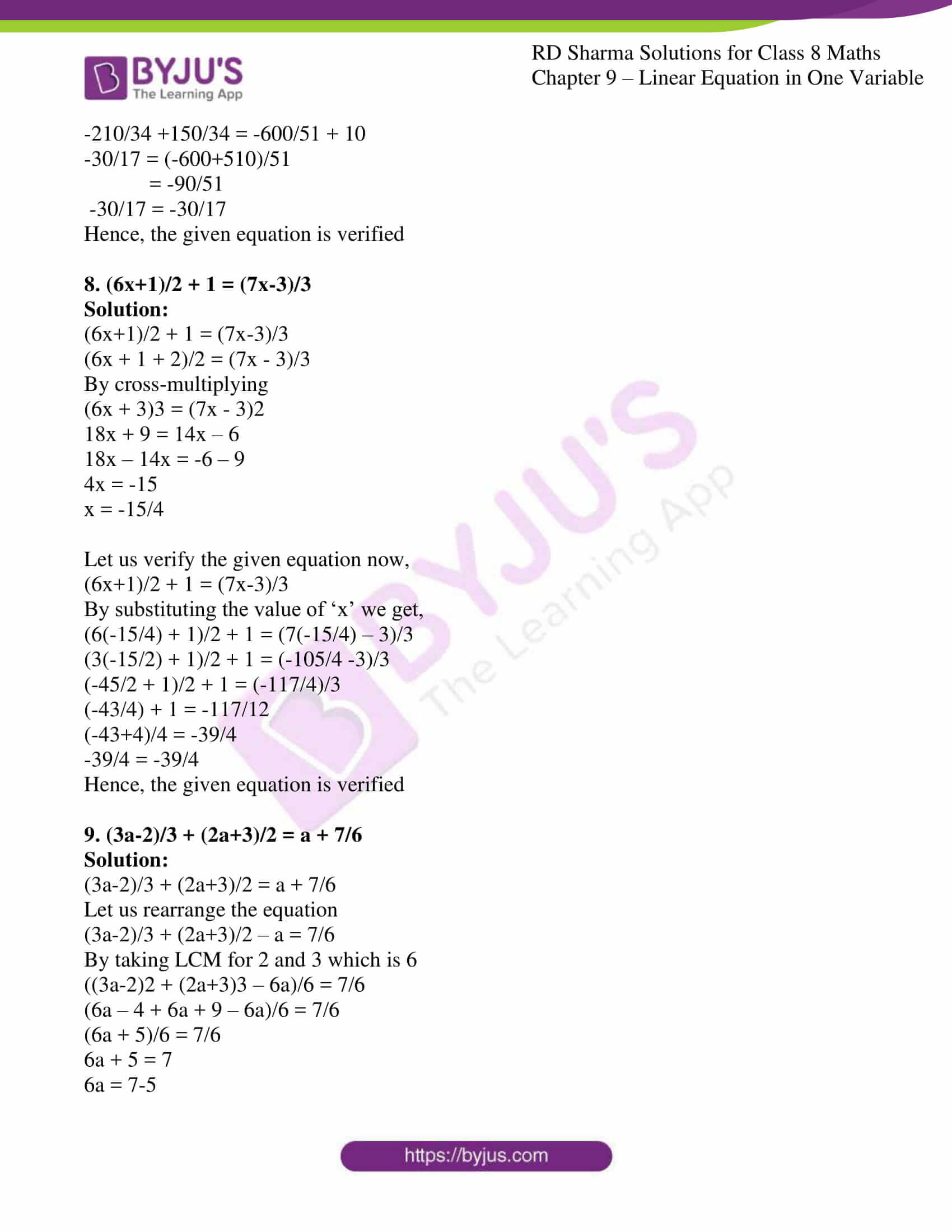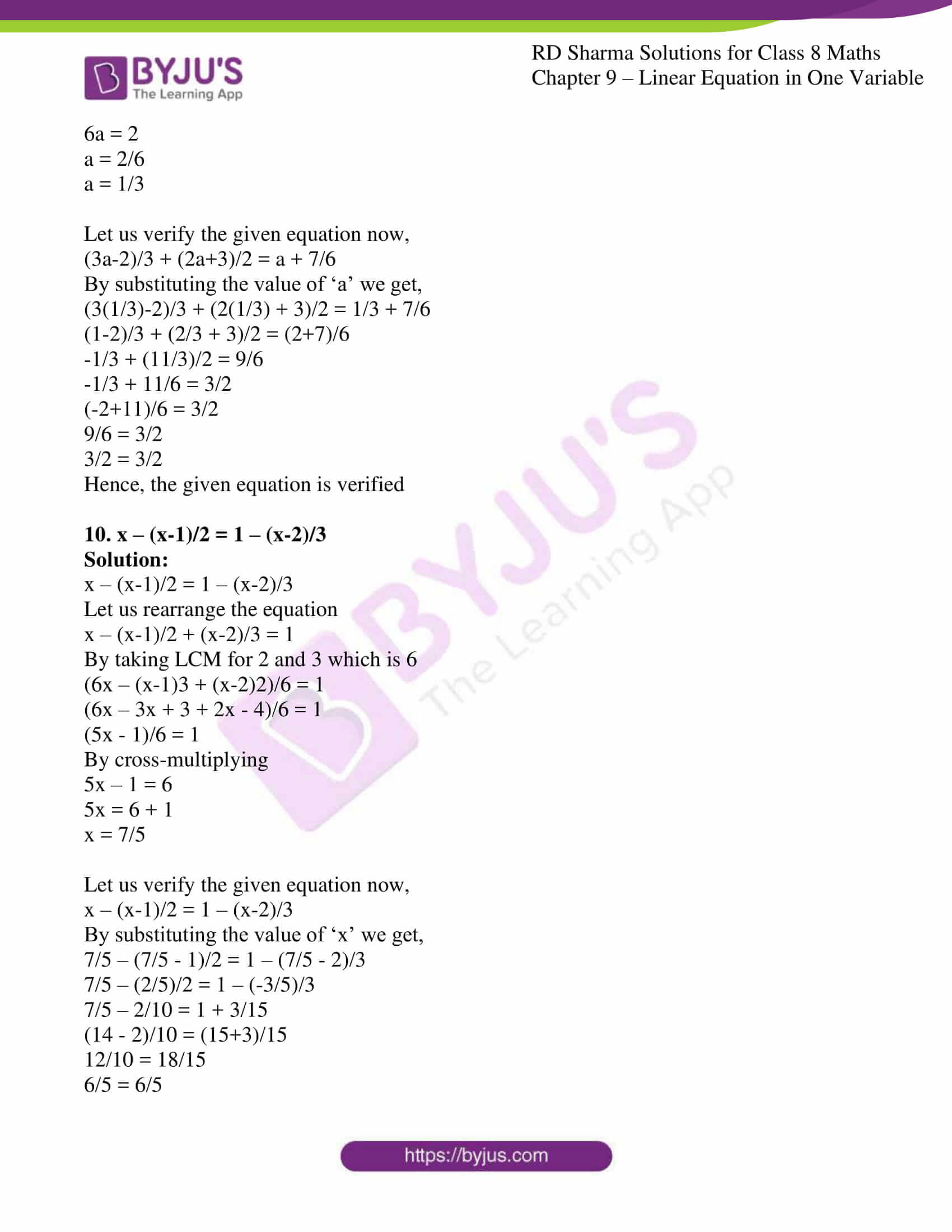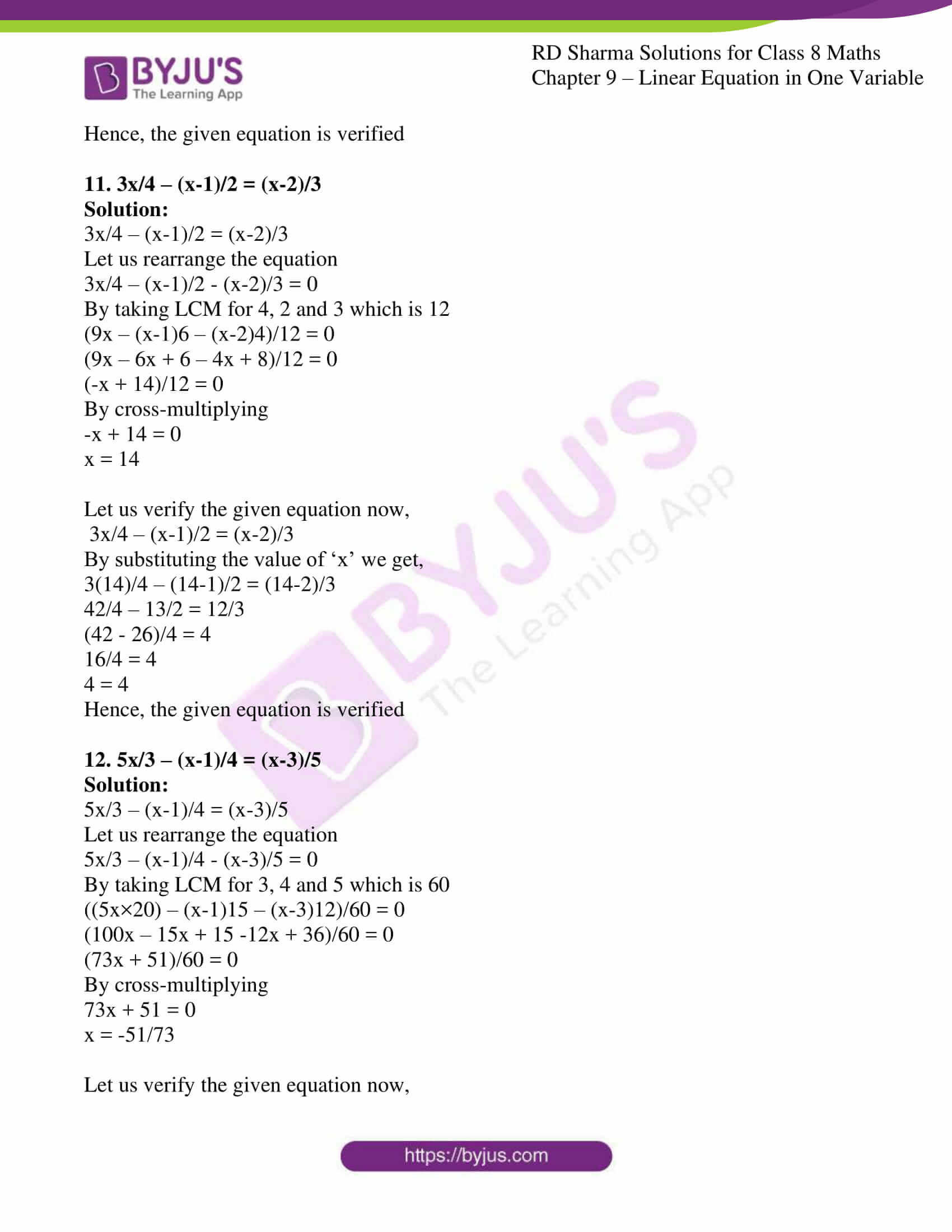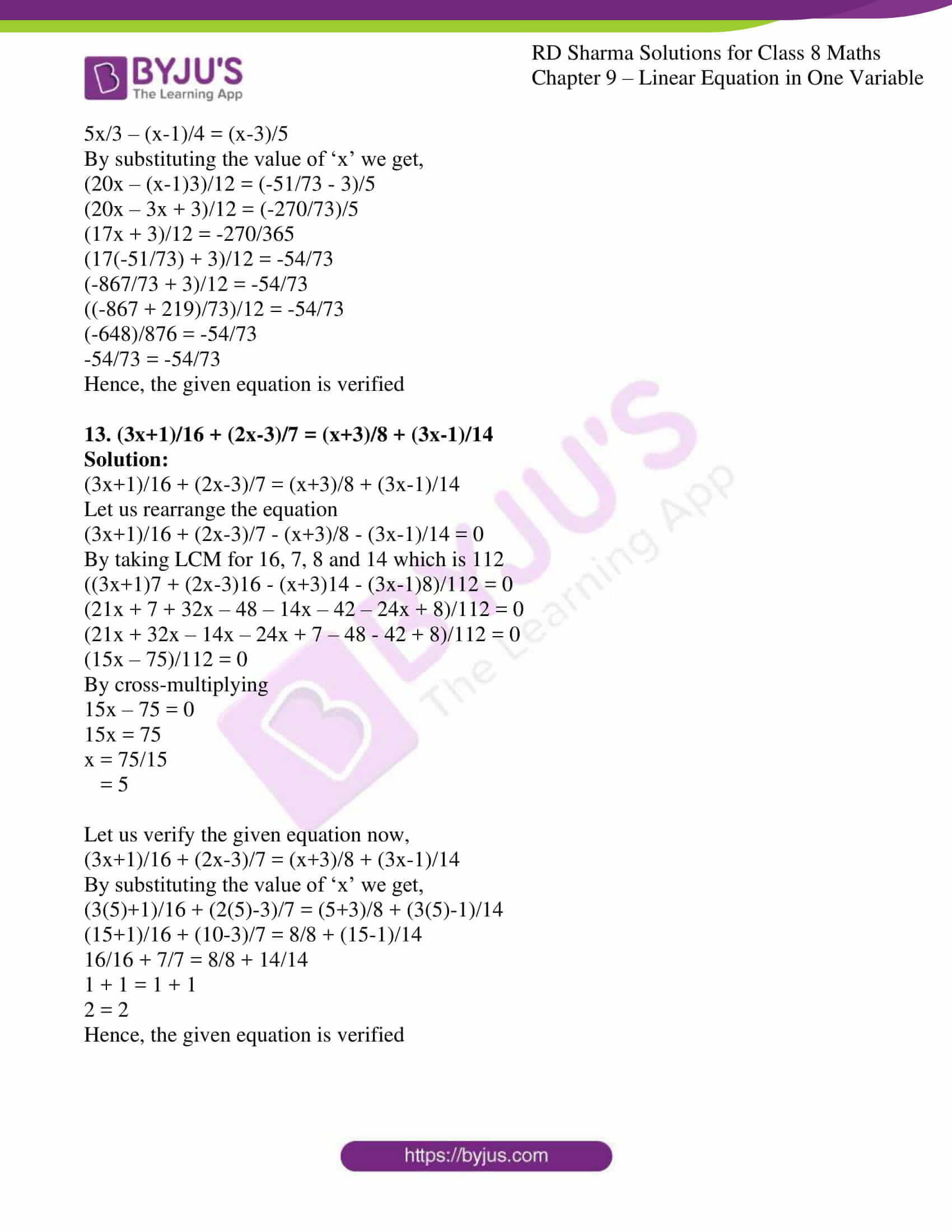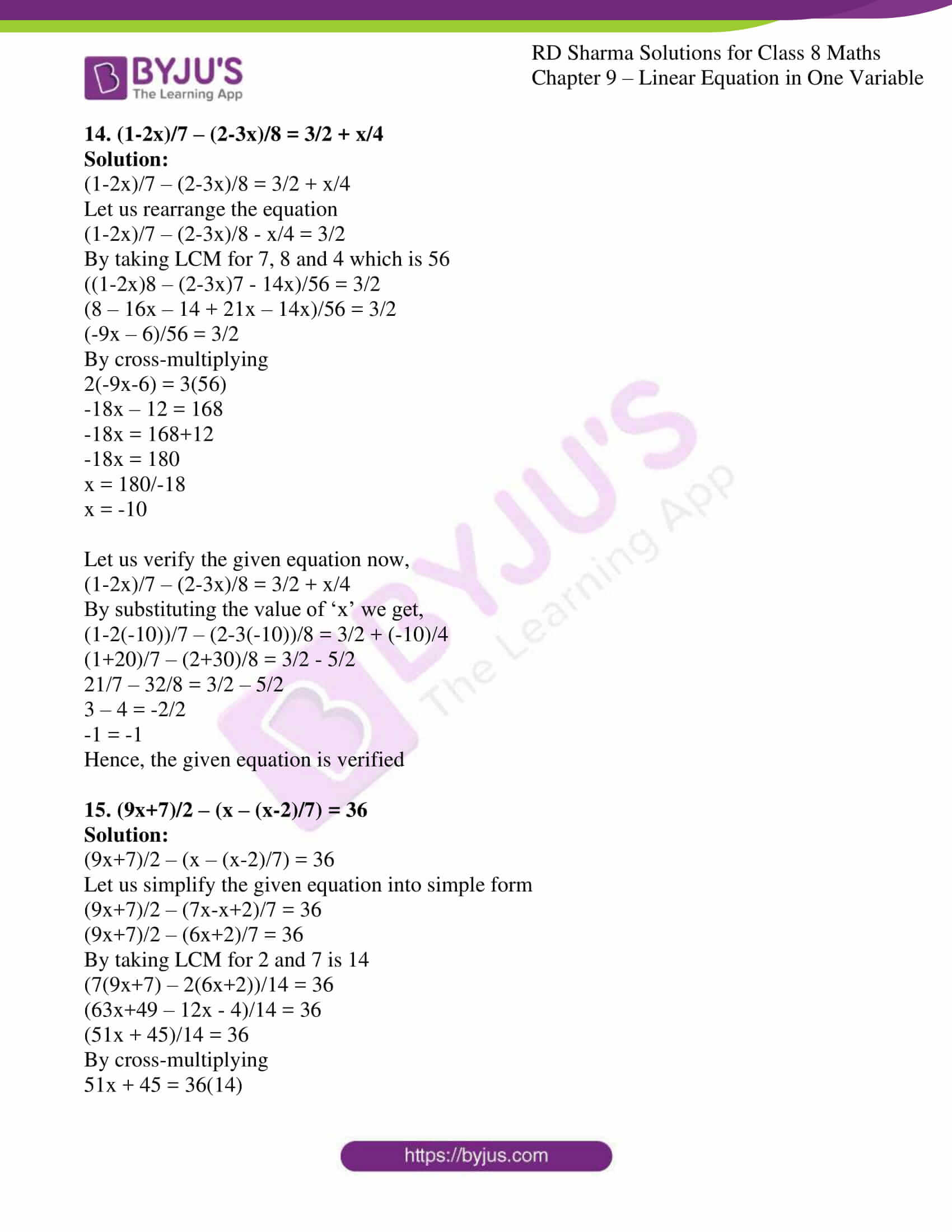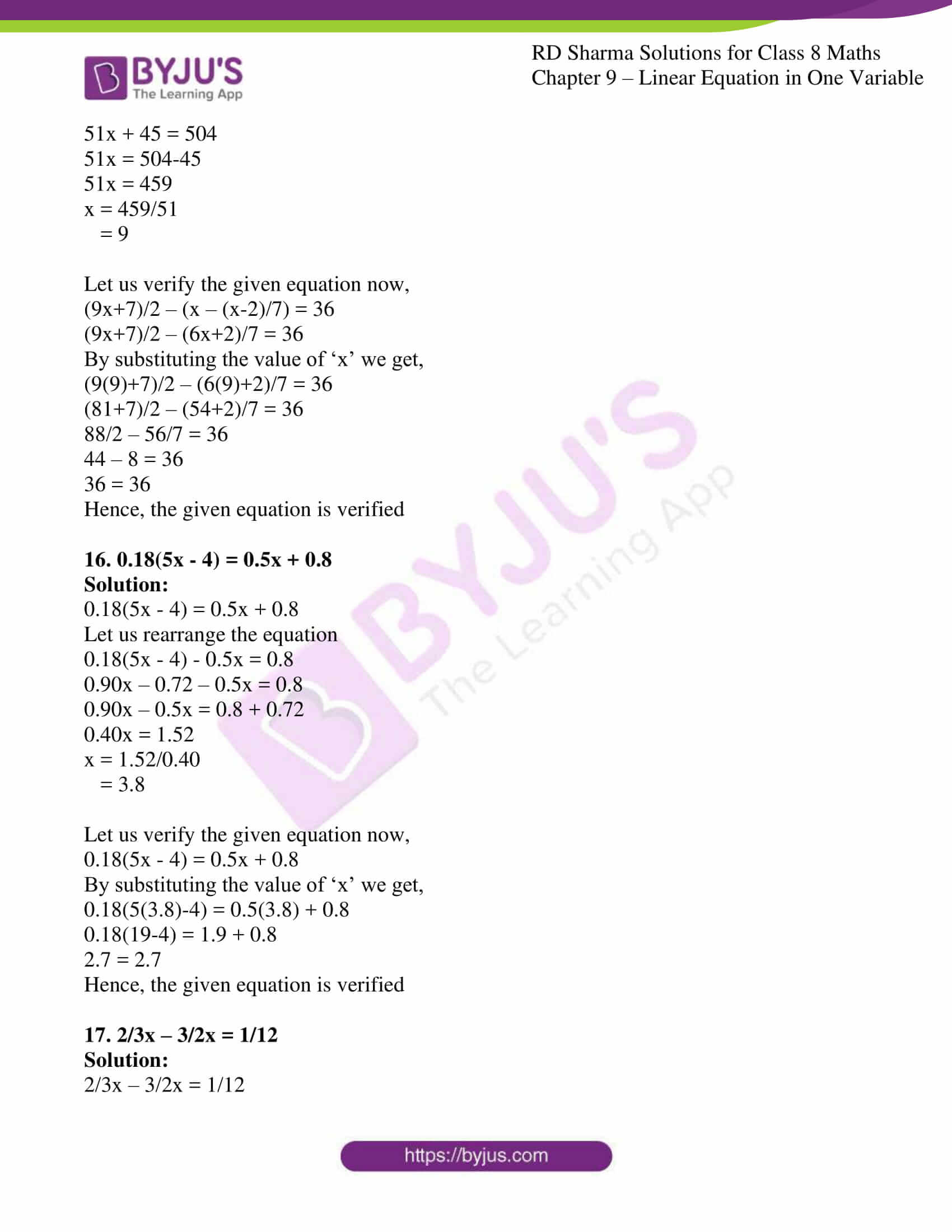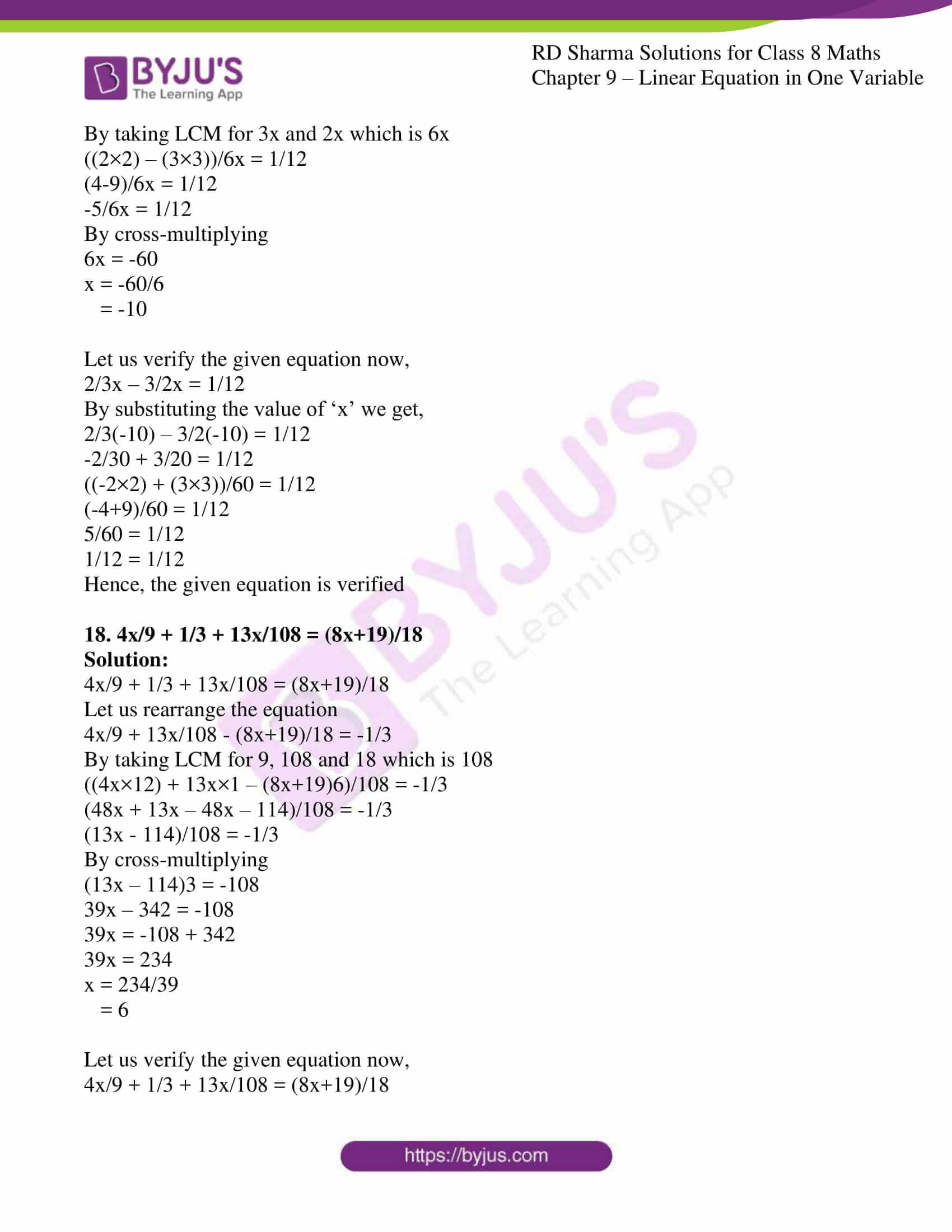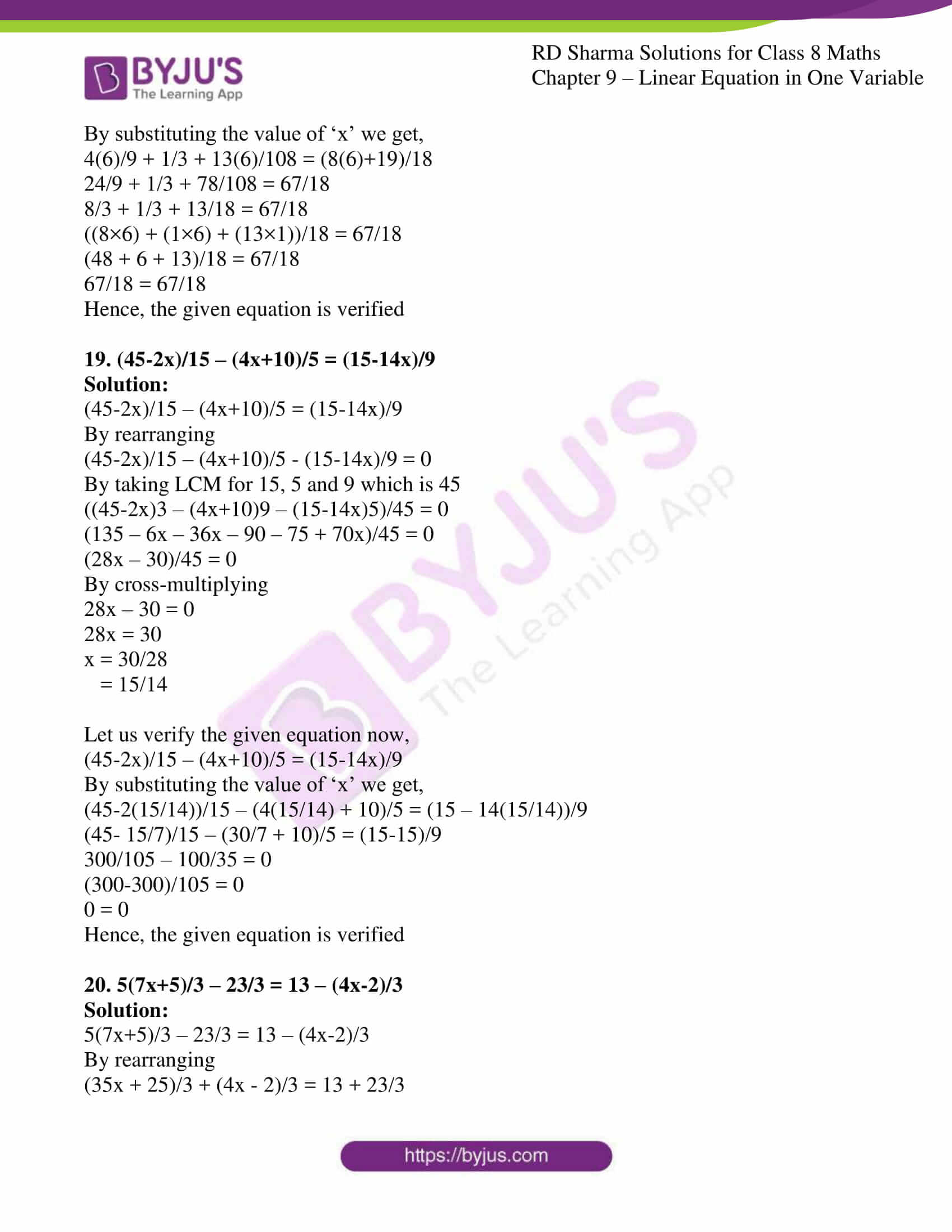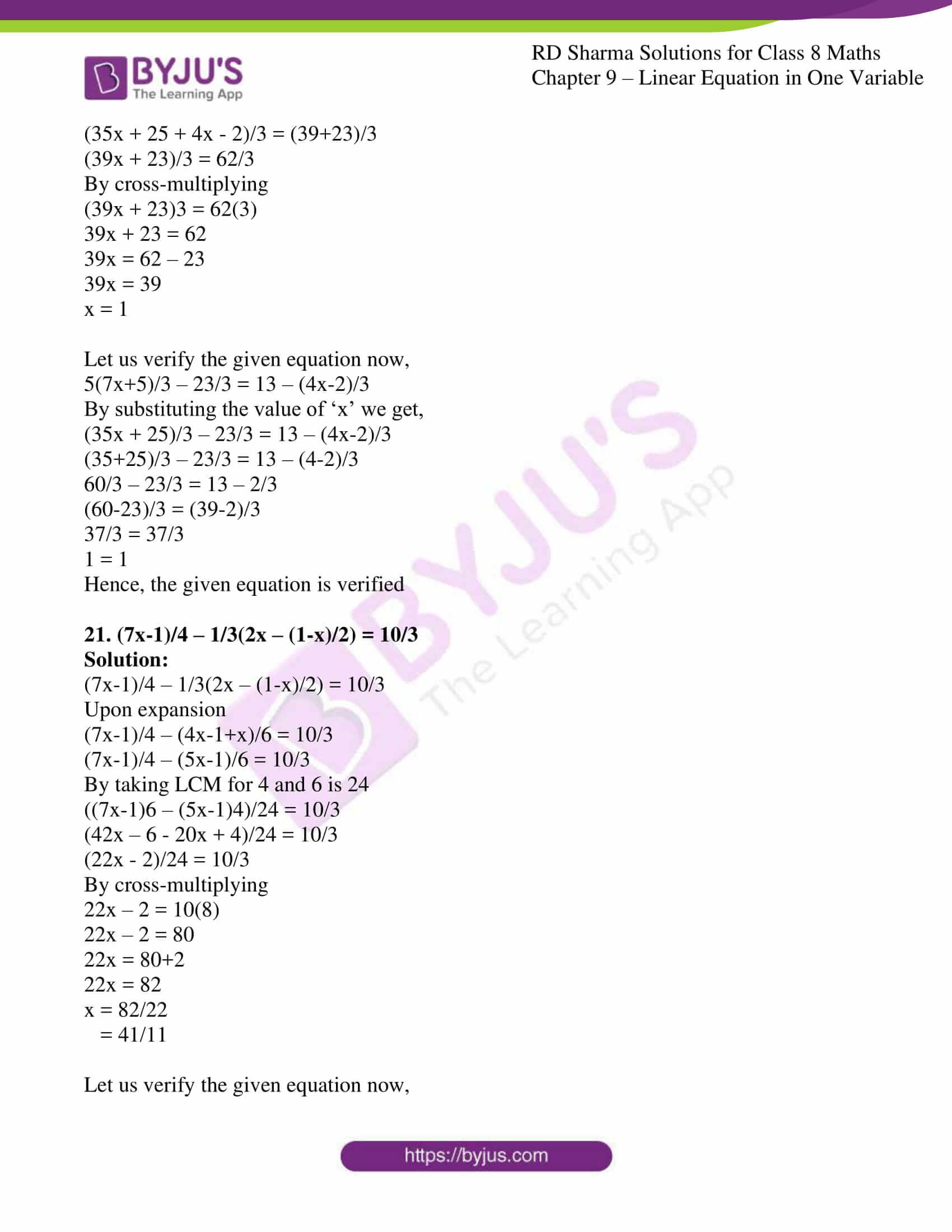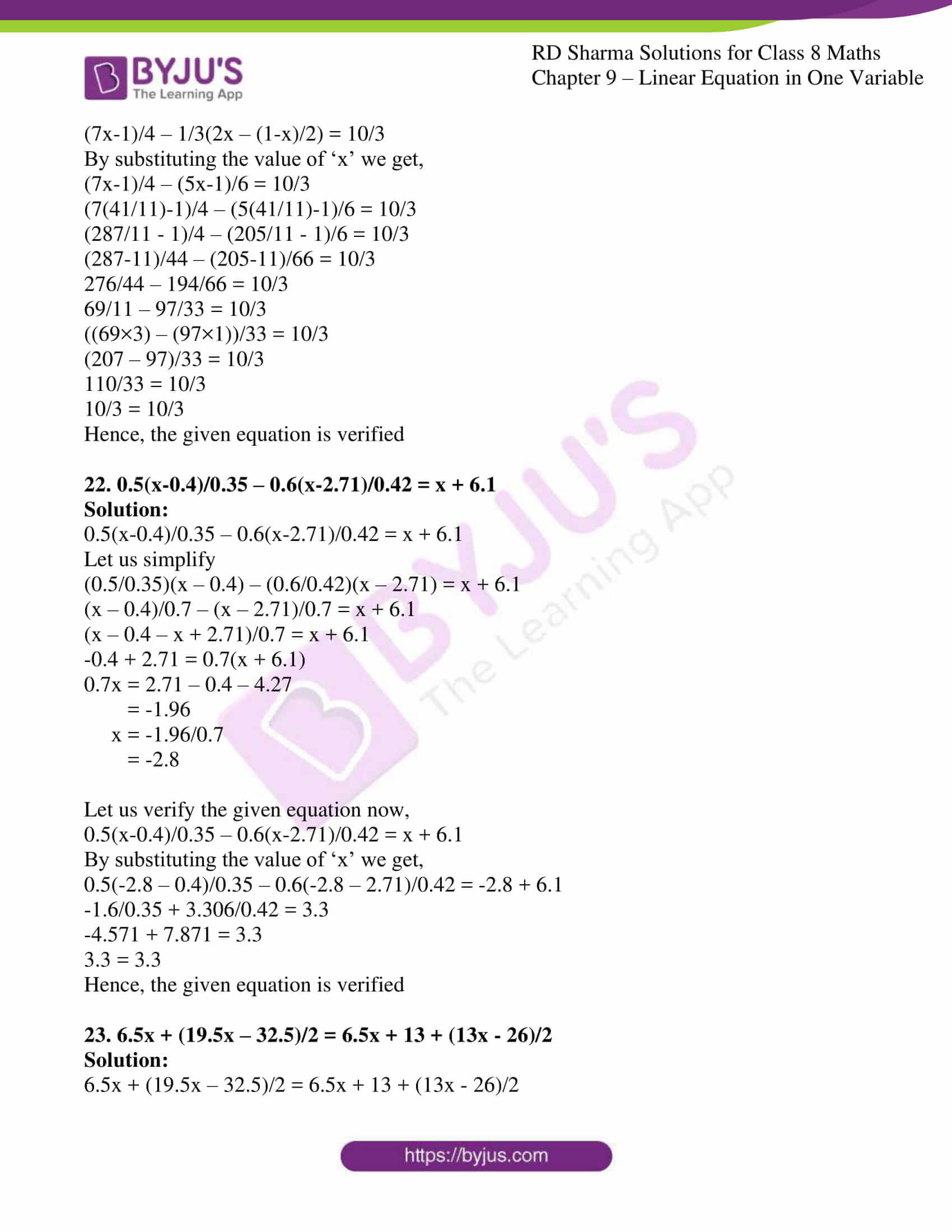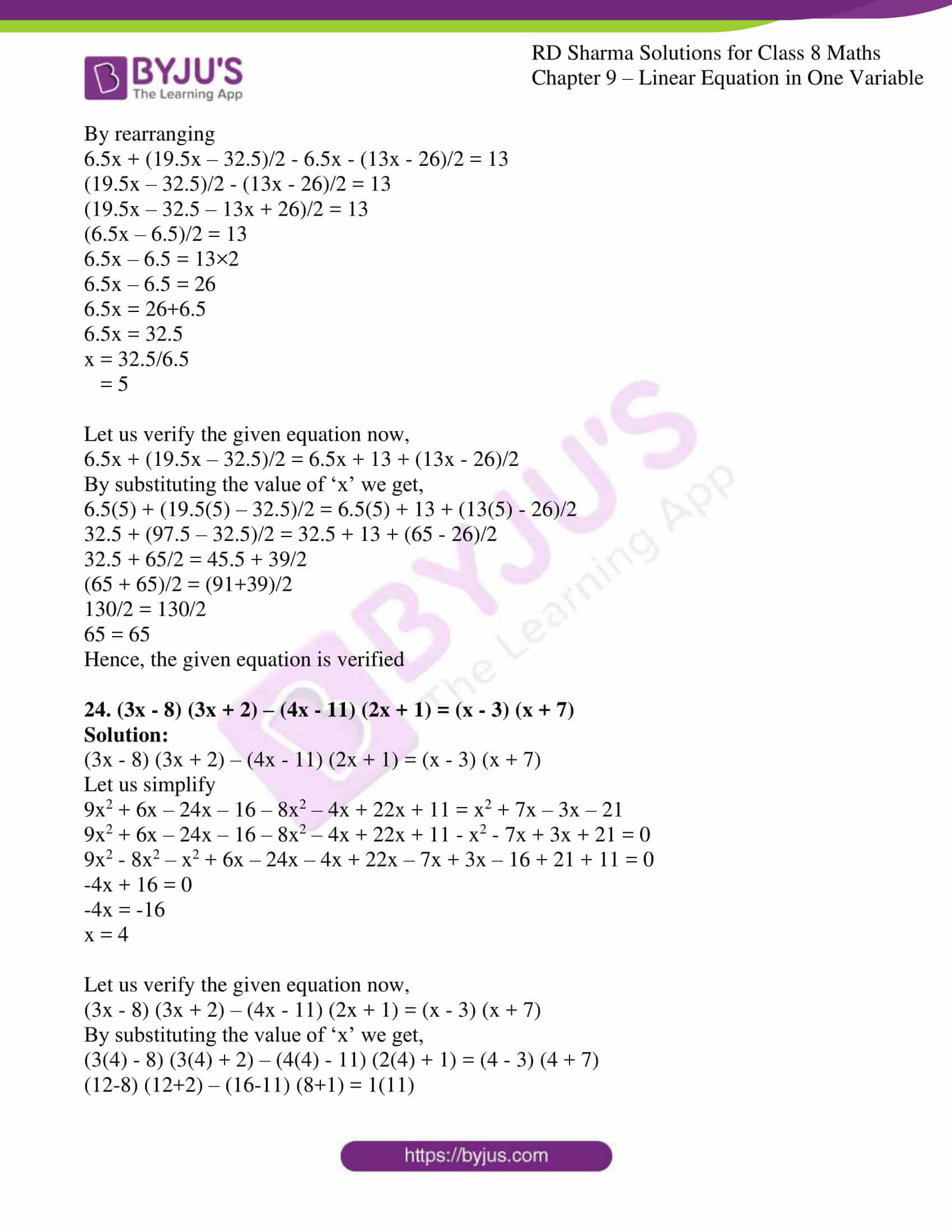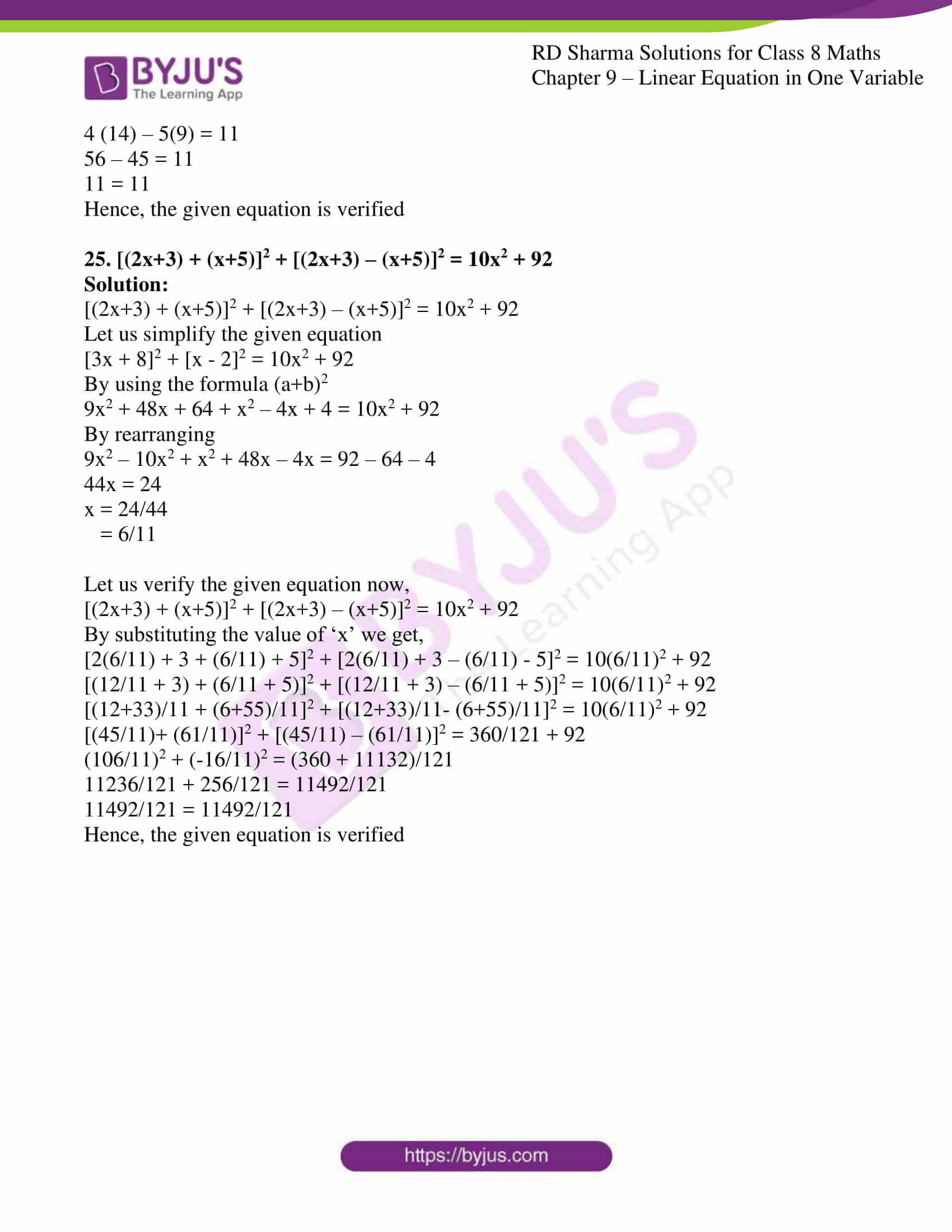### Access other Exercises of RD Sharma Solutions for Class 8 Maths Chapter 9 Linear Equation in One Variable

Exercise 9.1 Solutions

Exercise 9.3 Solutions

Exercise 9.4 Solutions

### Access Answers to RD Sharma Solutions for Class 8 Maths Exercise 9.2 Chapter 9 Linear Equation in One Variable

Solve each of the following equations and also check your results in each case:

1. (2x+5)/3 = 3x – 10

Solution:

(2x+5)/3 = 3x – 10

Let us simplify,

(2x+5)/3 – 3x = – 10

By taking LCM

(2x + 5 – 9x)/3 = -10

(-7x + 5)/3 = -10

By using cross-multiplication we get,

-7x + 5 = -30

-7x = -30 – 5

-7x = -35

x = -35/-7

= 5

Let us verify the given equation now,

(2x+5)/3 = 3x – 10

By substituting the value of ‘x’ we get,

(2×5 + 5)/3 = 3(5) – 10

(10+5)/3 = 15-10

15/3 = 5

5 = 5

Hence, the given equation is verified

2. (a-8)/3 = (a-3)/2

Solution:

(a-8)/3 = (a-3)/2

By using cross-multiplication we get,

(a-8)2 = (a-3)3

2a – 16 = 3a – 9

2a – 3a = -9 + 16

-a = 7

a = -7

Let us verify the given equation now,

(a-8)/3 = (a-3)/2

By substituting the value of ‘a’ we get,

(-7 – 8)/3 = (-7 – 3)/2

-15/3 = -10/2

-5 = -5

Hence, the given equation is verified

3. (7y + 2)/5 = (6y – 5)/11

Solution:

(7y + 2)/5 = (6y – 5)/11

By using cross-multiplication we get,

(7y + 2)11 = (6y – 5)5

77y + 22 = 30y – 25

77y – 30y = -25 – 22

47y = -47

y = -47/47

y = -1

Let us verify the given equation now,

(7y + 2)/5 = (6y – 5)/11

By substituting the value of ‘y’ we get,

(7(-1) + 2)/5 = (6(-1) – 5)/11

(-7 + 2)/5 = (-6 – 5)/11

-5/5 = -11/11

-1 = -1

Hence, the given equation is verified

4. x – 2x + 2 – 16/3x + 5 = 3 – 7/2x

Solution:

x – 2x + 2 – 16/3x + 5 = 3 – 7/2x

Let us rearrange the equation

x – 2x – 16x/3 + 7x/2 = 3 – 2 – 5

By taking LCM for 2 and 3 which is 6

(6x – 12x – 32x + 21x)/6 = -4

-17x/6 = -4

By cross-multiplying

-17x = -4×6

-17x = -24

x = -24/-17

x = 24/17

Let us verify the given equation now,

x – 2x + 2 – 16/3x + 5 = 3 – 7/2x

By substituting the value of ‘x’ we get,

24/17 – 2(24/17) + 2 – (16/3)(24/17) + 5 = 3 – (7/2)(24/17)

24/17 – 48/17 + 2 – 384/51 + 5 = 3 – 168/34

By taking 51 and 34 as the LCM we get,

(72 – 144 + 102 – 384 + 255)/51 = (102 – 168)/34

-99/51 = -66/34

-33/17 = -33/17

Hence, the given equation is verified

5. 1/2x + 7x – 6 = 7x + 1/4

Solution:

1/2x + 7x – 6 = 7x + 1/4

Let us rearrange the equation

1/2x + 7x – 7x = 1/4 + 6 (by taking LCM)

1/2x = (1+ 24)/4

1/2x = 25/4

By cross-multiplying

4x = 25 × 2

4x = 50

x = 50/4

x = 25/2

Let us verify the given equation now,

1/2x + 7x – 6 = 7x + 1/4

By substituting the value of ‘x’ we get,

(1/2) (25/2) + 7(25/2) – 6 = 7(25/2) + 1/4

25/4 + 175/2 – 6 = 175/2 + 1/4

By taking LCM for 4 and 2 is 4

(25 + 350 – 24)/4 = (350+1)/4

351/4 = 351/4

Hence, the given equation is verified

6. 3/4x + 4x = 7/8 + 6x – 6

Solution:

3/4x + 4x = 7/8 + 6x – 6

Let us rearrange the equation

3/4x + 4x – 6x = 7/8 – 6

By taking 4 and 8 as LCM

(3x + 16x – 24x)/4 = (7 – 48)/8

-5x/4 = -41/8

By cross-multiplying

-5x(8) = -41(4)

-40x = -164

x = -164/-40

= 82/20

= 41/10

Let us verify the given equation now,

3/4x + 4x = 7/8 + 6x – 6

By substituting the value of ‘x’ we get,

(3/4)(41/10) + 4(41/10) = 7/8 + 6(41/10) – 6

123/40 + 164/10 = 7/8 + 246/10 – 6

(123 + 656)/40 = (70 + 1968 – 480)/80

779/40 = 1558/80

779/40 = 779/40

Hence, the given equation is verified

7. 7x/2 – 5x/2 = 20x/3 + 10

Solution:

7x/2 – 5x/2 = 20x/3 + 10

Let us rearrange the equation

7x/2 – 5x/2 – 20x/3 = 10

By taking LCM for 2 and 3 is 6

(21x – 15x – 40x)/6 = 10

-34x/6 = 10

By cross-multiplying

-34x = 60

x = 60/-34

= -30/17

Let us verify the given equation now,

7x/2 – 5x/2 = 20x/3 + 10

By substituting the value of ‘x’ we get,

(7-/2)(-30/17) – (5/2)(-30/17) = (20/3)(-30/17) + 10

-210/34 +150/34 = -600/51 + 10

-30/17 = (-600+510)/51

= -90/51

-30/17 = -30/17

Hence, the given equation is verified

8. (6x+1)/2 + 1 = (7x-3)/3

Solution:

(6x+1)/2 + 1 = (7x-3)/3

(6x + 1 + 2)/2 = (7x – 3)/3

By cross-multiplying

(6x + 3)3 = (7x – 3)2

18x + 9 = 14x – 6

18x – 14x = -6 – 9

4x = -15

x = -15/4

Let us verify the given equation now,

(6x+1)/2 + 1 = (7x-3)/3

By substituting the value of ‘x’ we get,

(6(-15/4) + 1)/2 + 1 = (7(-15/4) – 3)/3

(3(-15/2) + 1)/2 + 1 = (-105/4 -3)/3

(-45/2 + 1)/2 + 1 = (-117/4)/3

(-43/4) + 1 = -117/12

(-43+4)/4 = -39/4

-39/4 = -39/4

Hence, the given equation is verified

9. (3a-2)/3 + (2a+3)/2 = a + 7/6

Solution:

(3a-2)/3 + (2a+3)/2 = a + 7/6

Let us rearrange the equation

(3a-2)/3 + (2a+3)/2 – a = 7/6

By taking LCM for 2 and 3 which is 6

((3a-2)2 + (2a+3)3 – 6a)/6 = 7/6

(6a – 4 + 6a + 9 – 6a)/6 = 7/6

(6a + 5)/6 = 7/6

6a + 5 = 7

6a = 7-5

6a = 2

a = 2/6

a = 1/3

Let us verify the given equation now,

(3a-2)/3 + (2a+3)/2 = a + 7/6

By substituting the value of ‘a’ we get,

(3(1/3)-2)/3 + (2(1/3) + 3)/2 = 1/3 + 7/6

(1-2)/3 + (2/3 + 3)/2 = (2+7)/6

-1/3 + (11/3)/2 = 9/6

-1/3 + 11/6 = 3/2

(-2+11)/6 = 3/2

9/6 = 3/2

3/2 = 3/2

Hence, the given equation is verified

10. x – (x-1)/2 = 1 – (x-2)/3

Solution:

x – (x-1)/2 = 1 – (x-2)/3

Let us rearrange the equation

x – (x-1)/2 + (x-2)/3 = 1

By taking LCM for 2 and 3 which is 6

(6x – (x-1)3 + (x-2)2)/6 = 1

(6x – 3x + 3 + 2x – 4)/6 = 1

(5x – 1)/6 = 1

By cross-multiplying

5x – 1 = 6

5x = 6 + 1

x = 7/5

Let us verify the given equation now,

x – (x-1)/2 = 1 – (x-2)/3

By substituting the value of ‘x’ we get,

7/5 – (7/5 – 1)/2 = 1 – (7/5 – 2)/3

7/5 – (2/5)/2 = 1 – (-3/5)/3

7/5 – 2/10 = 1 + 3/15

(14 – 2)/10 = (15+3)/15

12/10 = 18/15

6/5 = 6/5

Hence, the given equation is verified

11. 3x/4 – (x-1)/2 = (x-2)/3

Solution:

3x/4 – (x-1)/2 = (x-2)/3

Let us rearrange the equation

3x/4 – (x-1)/2 – (x-2)/3 = 0

By taking LCM for 4, 2 and 3 which is 12

(9x – (x-1)6 – (x-2)4)/12 = 0

(9x – 6x + 6 – 4x + 8)/12 = 0

(-x + 14)/12 = 0

By cross-multiplying

-x + 14 = 0

x = 14

Let us verify the given equation now,

3x/4 – (x-1)/2 = (x-2)/3

By substituting the value of ‘x’ we get,

3(14)/4 – (14-1)/2 = (14-2)/3

42/4 – 13/2 = 12/3

(42 – 26)/4 = 4

16/4 = 4

4 = 4

Hence, the given equation is verified

12. 5x/3 – (x-1)/4 = (x-3)/5

Solution:

5x/3 – (x-1)/4 = (x-3)/5

Let us rearrange the equation

5x/3 – (x-1)/4 – (x-3)/5 = 0

By taking LCM for 3, 4 and 5 which is 60

((5x×20) – (x-1)15 – (x-3)12)/60 = 0

(100x – 15x + 15 -12x + 36)/60 = 0

(73x + 51)/60 = 0

By cross-multiplying

73x + 51 = 0

x = -51/73

Let us verify the given equation now,

5x/3 – (x-1)/4 = (x-3)/5

By substituting the value of ‘x’ we get,

(20x – (x-1)3)/12 = (-51/73 – 3)/5

(20x – 3x + 3)/12 = (-270/73)/5

(17x + 3)/12 = -270/365

(17(-51/73) + 3)/12 = -54/73

(-867/73 + 3)/12 = -54/73

((-867 + 219)/73)/12 = -54/73

(-648)/876 = -54/73

-54/73 = -54/73

Hence, the given equation is verified

13. (3x+1)/16 + (2x-3)/7 = (x+3)/8 + (3x-1)/14

Solution:

(3x+1)/16 + (2x-3)/7 = (x+3)/8 + (3x-1)/14

Let us rearrange the equation

(3x+1)/16 + (2x-3)/7 – (x+3)/8 – (3x-1)/14 = 0

By taking LCM for 16, 7, 8 and 14 which is 112

((3x+1)7 + (2x-3)16 – (x+3)14 – (3x-1)8)/112 = 0

(21x + 7 + 32x – 48 – 14x – 42 – 24x + 8)/112 = 0

(21x + 32x – 14x – 24x + 7 – 48 – 42 + 8)/112 = 0

(15x – 75)/112 = 0

By cross-multiplying

15x – 75 = 0

15x = 75

x = 75/15

= 5

Let us verify the given equation now,

(3x+1)/16 + (2x-3)/7 = (x+3)/8 + (3x-1)/14

By substituting the value of ‘x’ we get,

(3(5)+1)/16 + (2(5)-3)/7 = (5+3)/8 + (3(5)-1)/14

(15+1)/16 + (10-3)/7 = 8/8 + (15-1)/14

16/16 + 7/7 = 8/8 + 14/14

1 + 1 = 1 + 1

2 = 2

Hence, the given equation is verified

14. (1-2x)/7 – (2-3x)/8 = 3/2 + x/4

Solution:

(1-2x)/7 – (2-3x)/8 = 3/2 + x/4

Let us rearrange the equation

(1-2x)/7 – (2-3x)/8 – x/4 = 3/2

By taking LCM for 7, 8 and 4 which is 56

((1-2x)8 – (2-3x)7 – 14x)/56 = 3/2

(8 – 16x – 14 + 21x – 14x)/56 = 3/2

(-9x – 6)/56 = 3/2

By cross-multiplying

2(-9x-6) = 3(56)

-18x – 12 = 168

-18x = 168+12

-18x = 180

x = 180/-18

x = -10

Let us verify the given equation now,

(1-2x)/7 – (2-3x)/8 = 3/2 + x/4

By substituting the value of ‘x’ we get,

(1-2(-10))/7 – (2-3(-10))/8 = 3/2 + (-10)/4

(1+20)/7 – (2+30)/8 = 3/2 – 5/2

21/7 – 32/8 = 3/2 – 5/2

3 – 4 = -2/2

-1 = -1

Hence, the given equation is verified

15. (9x+7)/2 – (x – (x-2)/7) = 36

Solution:

(9x+7)/2 – (x – (x-2)/7) = 36

Let us simplify the given equation into simple form

(9x+7)/2 – (7x-x+2)/7 = 36

(9x+7)/2 – (6x+2)/7 = 36

By taking LCM for 2 and 7 is 14

(7(9x+7) – 2(6x+2))/14 = 36

(63x+49 – 12x – 4)/14 = 36

(51x + 45)/14 = 36

By cross-multiplying

51x + 45 = 36(14)

51x + 45 = 504

51x = 504-45

51x = 459

x = 459/51

= 9

Let us verify the given equation now,

(9x+7)/2 – (x – (x-2)/7) = 36

(9x+7)/2 – (6x+2)/7 = 36

By substituting the value of ‘x’ we get,

(9(9)+7)/2 – (6(9)+2)/7 = 36

(81+7)/2 – (54+2)/7 = 36

88/2 – 56/7 = 36

44 – 8 = 36

36 = 36

Hence, the given equation is verified

16. 0.18(5x – 4) = 0.5x + 0.8

Solution:

0.18(5x – 4) = 0.5x + 0.8

Let us rearrange the equation

0.18(5x – 4) – 0.5x = 0.8

0.90x – 0.72 – 0.5x = 0.8

0.90x – 0.5x = 0.8 + 0.72

0.40x = 1.52

x = 1.52/0.40

= 3.8

Let us verify the given equation now,

0.18(5x – 4) = 0.5x + 0.8

By substituting the value of ‘x’ we get,

0.18(5(3.8)-4) = 0.5(3.8) + 0.8

0.18(19-4) = 1.9 + 0.8

2.7 = 2.7

Hence, the given equation is verified

17. 2/3x – 3/2x = 1/12

Solution:

2/3x – 3/2x = 1/12

By taking LCM for 3x and 2x which is 6x

((2×2) – (3×3))/6x = 1/12

(4-9)/6x = 1/12

-5/6x = 1/12

By cross-multiplying

6x = -60

x = -60/6

= -10

Let us verify the given equation now,

2/3x – 3/2x = 1/12

By substituting the value of ‘x’ we get,

2/3(-10) – 3/2(-10) = 1/12

-2/30 + 3/20 = 1/12

((-2×2) + (3×3))/60 = 1/12

(-4+9)/60 = 1/12

5/60 = 1/12

1/12 = 1/12

Hence, the given equation is verified

18. 4x/9 + 1/3 + 13x/108 = (8x+19)/18

Solution:

4x/9 + 1/3 + 13x/108 = (8x+19)/18

Let us rearrange the equation

4x/9 + 13x/108 – (8x+19)/18 = -1/3

By taking LCM for 9, 108 and 18 which is 108

((4x×12) + 13x×1 – (8x+19)6)/108 = -1/3

(48x + 13x – 48x – 114)/108 = -1/3

(13x – 114)/108 = -1/3

By cross-multiplying

(13x – 114)3 = -108

39x – 342 = -108

39x = -108 + 342

39x = 234

x = 234/39

= 6

Let us verify the given equation now,

4x/9 + 1/3 + 13x/108 = (8x+19)/18

By substituting the value of ‘x’ we get,

4(6)/9 + 1/3 + 13(6)/108 = (8(6)+19)/18

24/9 + 1/3 + 78/108 = 67/18

8/3 + 1/3 + 13/18 = 67/18

((8×6) + (1×6) + (13×1))/18 = 67/18

(48 + 6 + 13)/18 = 67/18

67/18 = 67/18

Hence, the given equation is verified

19. (45-2x)/15 – (4x+10)/5 = (15-14x)/9

Solution:

(45-2x)/15 – (4x+10)/5 = (15-14x)/9

By rearranging

(45-2x)/15 – (4x+10)/5 – (15-14x)/9 = 0

By taking LCM for 15, 5 and 9 which is 45

((45-2x)3 – (4x+10)9 – (15-14x)5)/45 = 0

(135 – 6x – 36x – 90 – 75 + 70x)/45 = 0

(28x – 30)/45 = 0

By cross-multiplying

28x – 30 = 0

28x = 30

x = 30/28

= 15/14

Let us verify the given equation now,

(45-2x)/15 – (4x+10)/5 = (15-14x)/9

By substituting the value of ‘x’ we get,

(45-2(15/14))/15 – (4(15/14) + 10)/5 = (15 – 14(15/14))/9

(45- 15/7)/15 – (30/7 + 10)/5 = (15-15)/9

300/105 – 100/35 = 0

(300-300)/105 = 0

0 = 0

Hence, the given equation is verified

20. 5(7x+5)/3 – 23/3 = 13 – (4x-2)/3

Solution:

5(7x+5)/3 – 23/3 = 13 – (4x-2)/3

By rearranging

(35x + 25)/3 + (4x – 2)/3 = 13 + 23/3

(35x + 25 + 4x – 2)/3 = (39+23)/3

(39x + 23)/3 = 62/3

By cross-multiplying

(39x + 23)3 = 62(3)

39x + 23 = 62

39x = 62 – 23

39x = 39

x = 1

Let us verify the given equation now,

5(7x+5)/3 – 23/3 = 13 – (4x-2)/3

By substituting the value of ‘x’ we get,

(35x + 25)/3 – 23/3 = 13 – (4x-2)/3

(35+25)/3 – 23/3 = 13 – (4-2)/3

60/3 – 23/3 = 13 – 2/3

(60-23)/3 = (39-2)/3

37/3 = 37/3

1 = 1

Hence, the given equation is verified

21. (7x-1)/4 – 1/3(2x – (1-x)/2) = 10/3

Solution:

(7x-1)/4 – 1/3(2x – (1-x)/2) = 10/3

Upon expansion

(7x-1)/4 – (4x-1+x)/6 = 10/3

(7x-1)/4 – (5x-1)/6 = 10/3

By taking LCM for 4 and 6 is 24

((7x-1)6 – (5x-1)4)/24 = 10/3

(42x – 6 – 20x + 4)/24 = 10/3

(22x – 2)/24 = 10/3

By cross-multiplying

22x – 2 = 10(8)

22x – 2 = 80

22x = 80+2

22x = 82

x = 82/22

= 41/11

Let us verify the given equation now,

(7x-1)/4 – 1/3(2x – (1-x)/2) = 10/3

By substituting the value of ‘x’ we get,

(7x-1)/4 – (5x-1)/6 = 10/3

(7(41/11)-1)/4 – (5(41/11)-1)/6 = 10/3

(287/11 – 1)/4 – (205/11 – 1)/6 = 10/3

(287-11)/44 – (205-11)/66 = 10/3

276/44 – 194/66 = 10/3

69/11 – 97/33 = 10/3

((69×3) – (97×1))/33 = 10/3

(207 – 97)/33 = 10/3

110/33 = 10/3

10/3 = 10/3

Hence, the given equation is verified

22. 0.5(x-0.4)/0.35 – 0.6(x-2.71)/0.42 = x + 6.1

Solution:

0.5(x-0.4)/0.35 – 0.6(x-2.71)/0.42 = x + 6.1

Let us simplify

(0.5/0.35)(x – 0.4) – (0.6/0.42)(x – 2.71) = x + 6.1

(x – 0.4)/0.7 – (x – 2.71)/0.7 = x + 6.1

(x – 0.4 – x + 2.71)/0.7 = x + 6.1

-0.4 + 2.71 = 0.7(x + 6.1)

0.7x = 2.71 – 0.4 – 4.27

= -1.96

x = -1.96/0.7

= -2.8

Let us verify the given equation now,

0.5(x-0.4)/0.35 – 0.6(x-2.71)/0.42 = x + 6.1

By substituting the value of ‘x’ we get,

0.5(-2.8 – 0.4)/0.35 – 0.6(-2.8 – 2.71)/0.42 = -2.8 + 6.1

-1.6/0.35 + 3.306/0.42 = 3.3

-4.571 + 7.871 = 3.3

3.3 = 3.3

Hence, the given equation is verified

23. 6.5x + (19.5x – 32.5)/2 = 6.5x + 13 + (13x – 26)/2

Solution:

6.5x + (19.5x – 32.5)/2 = 6.5x + 13 + (13x – 26)/2

By rearranging

6.5x + (19.5x – 32.5)/2 – 6.5x – (13x – 26)/2 = 13

(19.5x – 32.5)/2 – (13x – 26)/2 = 13

(19.5x – 32.5 – 13x + 26)/2 = 13

(6.5x – 6.5)/2 = 13

6.5x – 6.5 = 13×2

6.5x – 6.5 = 26

6.5x = 26+6.5

6.5x = 32.5

x = 32.5/6.5

= 5

Let us verify the given equation now,

6.5x + (19.5x – 32.5)/2 = 6.5x + 13 + (13x – 26)/2

By substituting the value of ‘x’ we get,

6.5(5) + (19.5(5) – 32.5)/2 = 6.5(5) + 13 + (13(5) – 26)/2

32.5 + (97.5 – 32.5)/2 = 32.5 + 13 + (65 – 26)/2

32.5 + 65/2 = 45.5 + 39/2

(65 + 65)/2 = (91+39)/2

130/2 = 130/2

65 = 65

Hence, the given equation is verified

24. (3x – 8) (3x + 2) – (4x – 11) (2x + 1) = (x – 3) (x + 7)

Solution:

(3x – 8) (3x + 2) – (4x – 11) (2x + 1) = (x – 3) (x + 7)

Let us simplify

9x2 + 6x – 24x – 16 – 8x2 – 4x + 22x + 11 = x2 + 7x – 3x – 21

9x2 + 6x – 24x – 16 – 8x2 – 4x + 22x + 11 – x2 – 7x + 3x + 21 = 0

9x2 – 8x2 – x2 + 6x – 24x – 4x + 22x – 7x + 3x – 16 + 21 + 11 = 0

-4x + 16 = 0

-4x = -16

x = 4

Let us verify the given equation now,

(3x – 8) (3x + 2) – (4x – 11) (2x + 1) = (x – 3) (x + 7)

By substituting the value of ‘x’ we get,

(3(4) – 8) (3(4) + 2) – (4(4) – 11) (2(4) + 1) = (4 – 3) (4 + 7)

(12-8) (12+2) – (16-11) (8+1) = 1(11)

4 (14) – 5(9) = 11

56 – 45 = 11

11 = 11

Hence, the given equation is verified

25. [(2x+3) + (x+5)]2 + [(2x+3) – (x+5)]2 = 10x2 + 92

Solution:

[(2x+3) + (x+5)]2 + [(2x+3) – (x+5)]2 = 10x2 + 92

Let us simplify the given equation

[3x + 8]2 + [x – 2]2 = 10x2 + 92

By using the formula (a+b)2

9x2 + 48x + 64 + x2 – 4x + 4 = 10x2 + 92

By rearranging

9x2 – 10x2 + x2 + 48x – 4x = 92 – 64 – 4

44x = 24

x = 24/44

= 6/11

Let us verify the given equation now,

[(2x+3) + (x+5)]2 + [(2x+3) – (x+5)]2 = 10x2 + 92

By substituting the value of ‘x’ we get,

[2(6/11) + 3 + (6/11) + 5]2 + [2(6/11) + 3 – (6/11) – 5]2 = 10(6/11)2 + 92

[(12/11 + 3) + (6/11 + 5)]2 + [(12/11 + 3) – (6/11 + 5)]2 = 10(6/11)2 + 92

[(12+33)/11 + (6+55)/11]2 + [(12+33)/11- (6+55)/11]2 = 10(6/11)2 + 92

[(45/11)+ (61/11)]2 + [(45/11) – (61/11)]2 = 360/121 + 92

(106/11)2 + (-16/11)2 = (360 + 11132)/121

11236/121 + 256/121 = 11492/121

11492/121 = 11492/121

Hence, the given equation is verified